Edges:The most common type of edge detection process uses a gradient operator, of which there have been several variations. Mathematically, for an image function, f(x,y), the gradient magnitude, g(x,y) and the gradient direction,(x,y) are computed asand,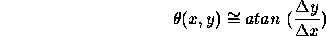where,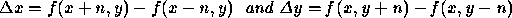and n is a small integer, usually unity. For example, the simplest implementation of this would be to convolve the following mask with the image data, aligning the mask with the x and y axes to compute the values of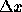and.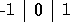Variations on this theme have included the Roberts, Prewitt and Sobel operators. The Sobel operator was by far the most extensively used until those based on the Gaussian function and its derivatives were recognised widely. In this case the masks are extended to a 3 by 3 neighbourhood, rather than the 3 by 1 neighbourhood given above. The x and y masks given below are first convolved with the image to compute the values ofand. Then the magnitude and angle of the edges are computed from these values and stored (usually) as two separate image frames.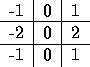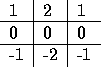Compared to a Laplacian edge operator, ( equivalent to edge enhancement but with a mean mask value of zero ), the Sobel operator has distinct advantages, although it is slightly more complex. It is less sensitive to isolated high intensity point variations since the local averaging over sets of three pixels tends to reduce this. In effect it is a ``small bar'' detector, rather than a point detector. Secondly, it gives an estimate of edge direction as well as edge magnitude at a point which is more informative and is of considerable use in later processing. A disadvantage of the Sobel edge detector is that the response to a step edge is present over either two or three pixels width, dependent on the precise position of the step relative to the pixel grid. This necessitates the use of post-processing in the form of edge ``thinning'' and ``thresholding'' in order to reduce the computational complexity of further processing. Figure 2 shows the magnitude response of the operator before any thresholding or thinning has occurred.

Although slightly more complex than the Laplacian operator, the Sobel operator is still relatively easy to implement in hardware form, most obviously by a pipeline approach. The first custom VLSI design of a Sobel operator was achieved in 1977, and several companies offer discrete versions of the operator in a simple plug-in assembly. In terms of accuracy, the Sobel operator may give an edge magnitude value to within 7% and an edge angle magnitude to within 2 degrees, ignoring the effects of quantisation errors, edge displacement from the centre of the pixel, electronic noise etc.Comments to: Sarah Price at ICBL.
(Last update: 4th July 1996)